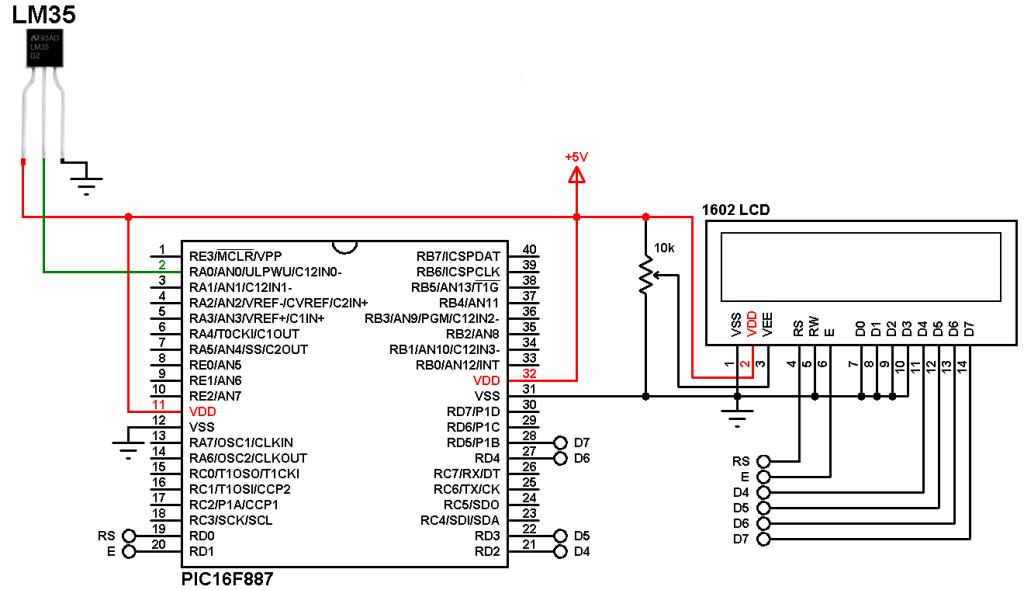# Interfacing PIC microcontroller with LM35 sensor – mikroC Projects

Interfacing the analog temperature sensor LM35 with PIC microcontroller is very easy, all what we need is an analog-to-digital converter (ADC) module associated with the PIC microcontroller. This page shows how to easily connect the LM35 sensor with PIC16F887 microcontroller and build a simple DIY thermometer. The compiler used in this project is mikroC PRO for PIC.

The LM35 temperature sensor is three pin device (VCC, OUT and GND) with an output voltage linearly related to Centigrade temperature (analog device). Since the LM35 output varies with dependent to the temperature we need an ADC (Analog-to-Digital Converter) module to measure this voltage. The ADC module converts analog data into digital data. The PIC16F887 microcontroller has 1 ADC module with 10-bit resolution and 14 channels.

The LM35 output has linear +10mV/°C scale factor means the following:
If the output voltage =   10mV —> temperature =   1°C
If the output voltage = 100mV —> temperature = 10°C
If the output voltage = 200mV —> temperature = 20°C
If the output voltage = 370mV —> temperature = 37°C
and so on.

Hardware Required:

• PIC16F887 microcontroller
• LM35 temperature sensor  — datasheet
• 1602 LCD screen
• 10k ohm variable resistor (or potentiometer)
• 5V voltage source
• Jumper wires

Interfacing PIC microcontroller with LM35 sensor circuit:
Example circuit diagram is shown below.(All grounded terminals are connected together)

The LM35 has 3 pins (from left to right):
Pin 1: VCC, connected to +5V
Pin 2: analog output, connected to RA0/AN0
Pin 3: GND (ground), connected to the ground of the circuit (0V)

The output pin of the LM335 sensor is connected to RA0/AN0 pin (analog pin 0) of the PIC16F887.

The LCD module is connected to PORTD pins with:
RS —> RD0
E  —> RD1
D4 —> RD2
D5 —> RD3
D6 —> RD4
D7 —> RD5

In this example the PIC16F887 microcontroller runs with its internal oscillator (@ 8MHz) and MCLR pin is configured as a digital input pin (configured in the software).

Interfacing PIC microcontroller with LM35 sensor mikroC code:
Reading voltage quantity using the ADC gives us a number between 0 and 1023 (10-bit resolution), 0V is represented by 0 and 5V is represented by 1023. Converting back the ADC digital value is easy and we can use the following equation for that conversion:
Multiplying the previous result by 100 (LM35 scale factor is 10mV/°C = 0.01V/°C) will gives the actual temperature: